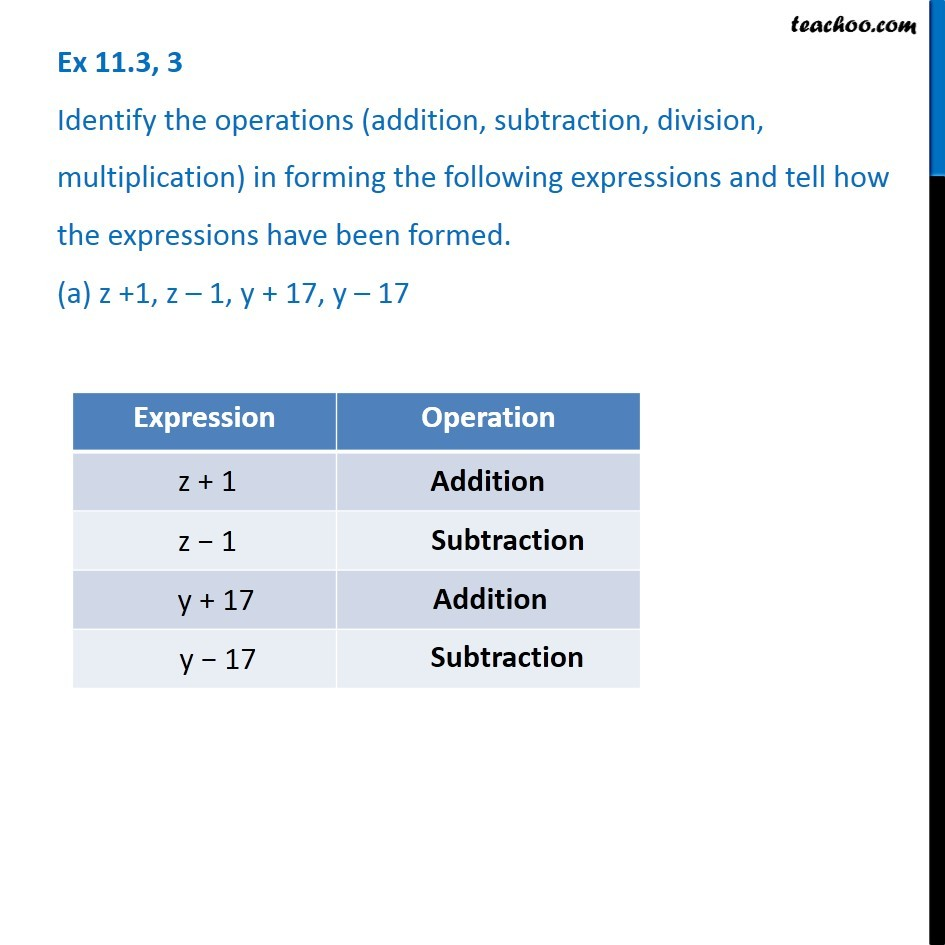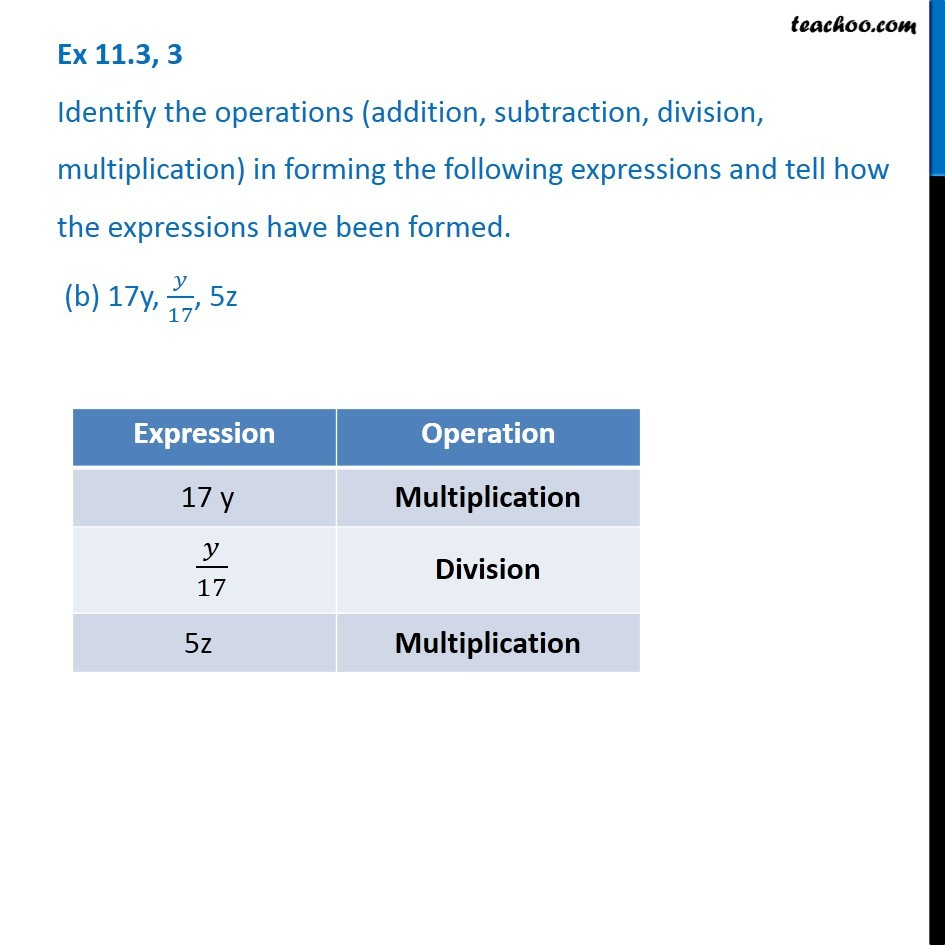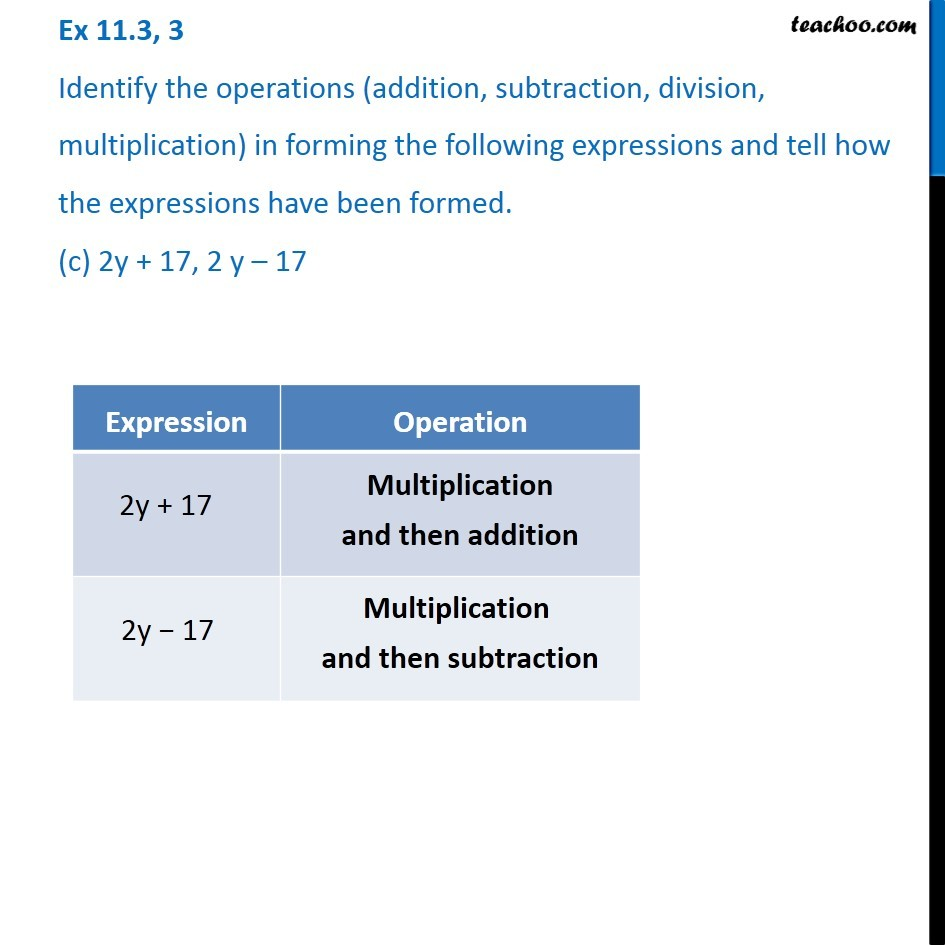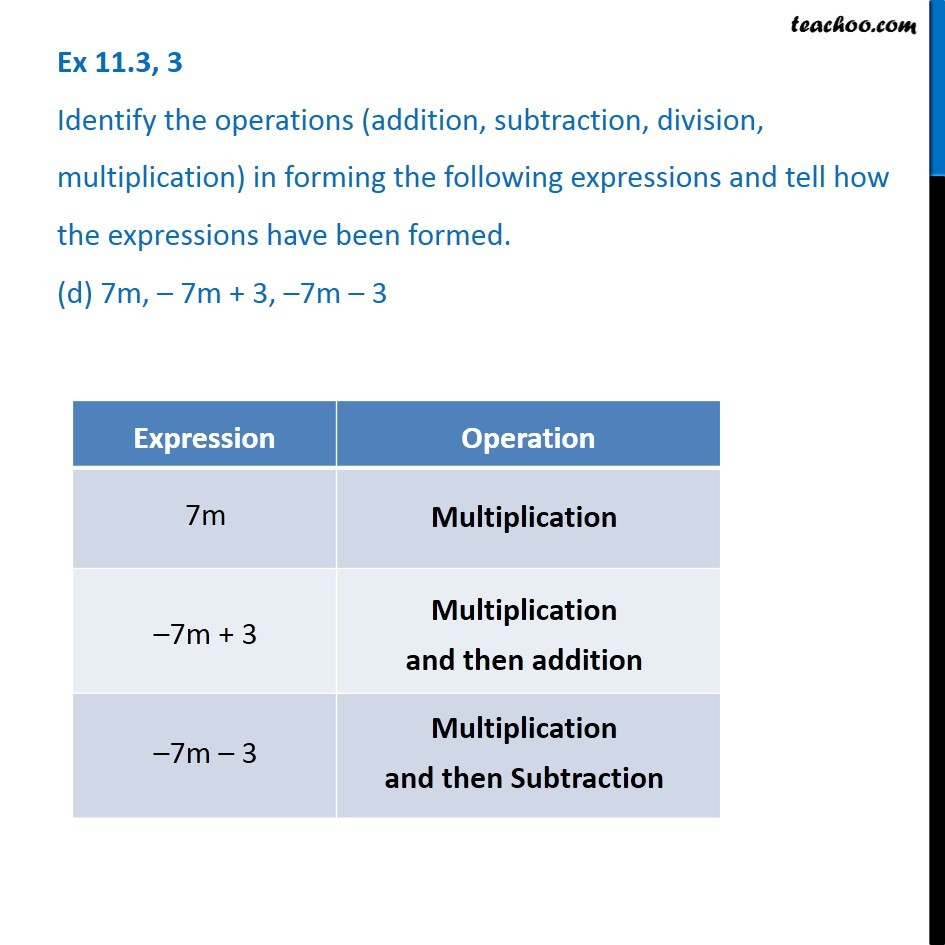Creating Basic Algebra Expressions - WorksheetLearn in your speed, with individual attention - Teachoo Maths 1-on-1 Class

### Transcript

Question 3 Identify the operations (addition, subtraction, division, multiplication) in forming the following expressions and tell how the expressions have been formed. (a) z +1, z – 1, y + 17, y – 17 Question 3 Identify the operations (addition, subtraction, division, multiplication) in forming the following expressions and tell how the expressions have been formed. (b) 17y, 𝑦/17, 5z Question 3 Identify the operations (addition, subtraction, division, multiplication) in forming the following expressions and tell how the expressions have been formed. (b) 17y, 𝑦/17, 5z Question 3 Identify the operations (addition, subtraction, division, multiplication) in forming the following expressions and tell how the expressions have been formed. (d) 7m, – 7m + 3, –7m – 3